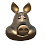### A 4D voronoi OSL shader for Blender Cycles

I took a post on Blender Artists as a challenge and created a 4D voronoi shader.

Blogspot won't show animated gifs but I put up a short sequence on PasteAll and if that doesn't work it is also on my site. In this animated gif, the fourth dimension (time) is animated from 0 to 1 in 50 frames.
The shader presented below is simple enough but convoluted because we cannot manipulate arrays like points or vectors in OSL but otherwise it is a straight forward extension of Blenders bundled voronoi implementation, especially because OSLs cellnoise function supports 4D noise out of the box (you can pass it a point and a float).
```#include "stdosl.h"

void cellnoise_color4d(float p, float c)
{
c = cellnoise(point(p,p,p),p);
c = cellnoise(point(p,p,p),p);
c = cellnoise(point(p,p,p),p);
c = cellnoise(point(p,p,p),p);
}

/* Voronoi 4D . we always use distance squared as the distance metric */

void voronoi4d(point p, float t, float da, point pa, float ta)
{
/* returns distances in da, point coords in pa and time coords in ta*/
int xx, yy, zz, tt, xi, yi, zi, ti;

float op = {p,p,p,t};

xi = (int)floor(p);
yi = (int)floor(p);
zi = (int)floor(p);
ti = (int)floor(t);

da = 1e10;
da = 1e10;
da = 1e10;
da = 1e10;

for (xx = xi - 1; xx <= xi + 1; xx++) {
for (yy = yi - 1; yy <= yi + 1; yy++) {
for (zz = zi - 1; zz <= zi + 1; zz++) {
for (tt = ti - 1; tt <= ti + 1; tt++) {
float ip = {xx, yy, zz, tt};
float vp;
cellnoise_color4d(ip,vp);
float pd = { op - (vp + ip),
op - (vp + ip),
op - (vp + ip),
op - (vp + ip)};
// always distance squared
float d = pd*pd+pd*pd+pd*pd+pd*pd;

vp += xx;
vp += yy;
vp += zz;
vp += tt;

if (d < da) {
da = da;
da = da;
da = da;
da = d;

pa = pa; ta = ta;
pa = pa; ta = ta;
pa = pa; ta = ta;
pa = point(vp,vp,vp); ta = vp;
}
else if (d < da) {
da = da;
da = da;
da = d;

pa = pa; ta = ta;
pa = pa; ta = ta;
pa = point(vp,vp,vp); ta = vp;
}
else if (d < da) {
da = da;
da = d;

pa = pa; ta = ta;
pa = point(vp,vp,vp); ta = vp;
}
else if (d < da) {
da = d;
pa = point(vp,vp,vp); ta = vp;
}
}
}
}
}
}

float Scale = 5.0,
point Vector = P,
float Time = 0,
output float Fac = 0.0,
output color Color = color(0.0, 0.0, 0.0))
{
point p = Vector;

/* compute distance and point coordinate of 4 nearest neighbours */
float da;
point pa;
float ta;

voronoi4d(p * Scale, Time * Scale, da, pa, ta);

Fac = fabs(da);
Color = color(Fac);
}```

## Example node setup

Straight forward enough but note the keyframed Time value.

1.2.